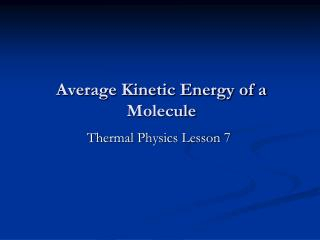DownloadDownload PresentationAverage Kinetic Energy of a Molecule

# Average Kinetic Energy of a Molecule

Télécharger la présentation## Average Kinetic Energy of a Molecule

- - - - - - - - - - - - - - - - - - - - - - - - - - - E N D - - - - - - - - - - - - - - - - - - - - - - - - - - -
##### Presentation Transcript

1. Average Kinetic Energy of a Molecule Thermal Physics Lesson 7

2. Learning Objectives • Derive an expression to calculate the average kinetic energy of a molecule.

3. Mean kinetic energy The mean kinetic energy of a molecule is the total kinetic energy of all the molecules/total number of molecules. The root mean square speed is defined as: …and so the mean kinetic energy of one molecule is gvien by…

4. Mean Kinetic Energy Note we have two equations with pV on the left hand side. (N=nNA) The Boltzmann constant, k, is defined as R/NA.

5. Mean Kinetic Energy Multiply both sides by 3/2. Mean kinetic energy of a molecule of an ideal gas. Kinetic energy of one mole of gas (because R=NAk) Kinetic energy of n moles of gas.